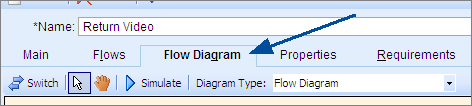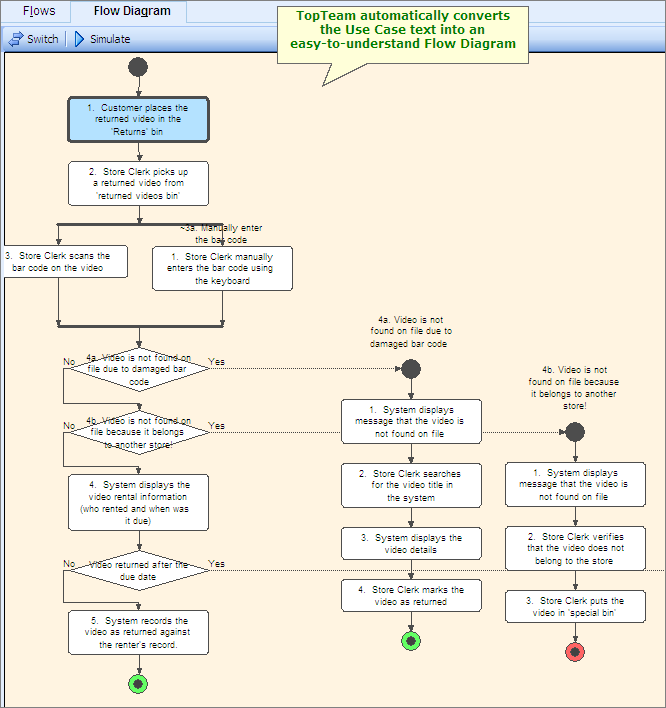﻿ Visualize Use Case text as a Flow Diagram
Tutorial: Defining Requirements with Use Cases using TopTeam

Visualize Use Case text using the automatically generated Flow Diagram

 Visualize Use Case text using the automatically generated Flow DiagramVisualize Use Case text using the automatically generated Flow DiagramOverview

TopTeam automatically converts the text of Use Case Flow-of-events into a Flow Diagram.

To visualize Use Cases using the Flow Diagram, follow these steps:

Go to the Flow Diagram Tab on the Use Case editor.As soon as you navigate to the Flow Diagram tab, TopTeam parses the text of the Use Case Flow and automatically generates a visual representation of the Use Case steps in the form of the Flow Diagram.Flow Diagram Toolbar

Use the functions available on the Flow Diagram toolbar (image below) to adjust the diagram for the best viewing experience.Flow DiagramStep Complete

You have now successfully visualized Use Case flow using the automatically generated Flow Diagram.

Proceed to the next step.